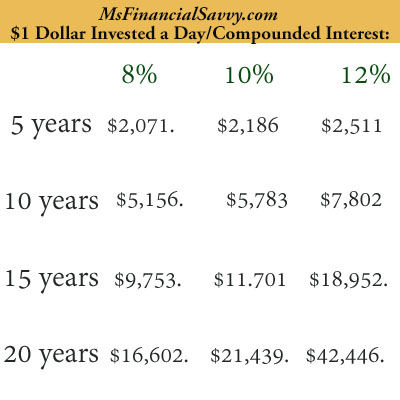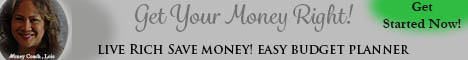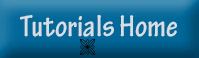# Compounded Interest

## Compounded Interest With Stocks and Mutual Funds

With stocks and mutual funds, long-term gains are often large due to compounded interest versus simple interest. With simple interest, you only get interested on an original principal. With investments such as stocks and mutual funds, you get interest on the principal and then interest on principal + interest, and so on, this is compounded interest.

Let’s demonstrate with one dollar. Suppose you save one dollar a day for several years at the following interest rates and number of years invested.

How much would you end up with continuously getting interest on interest on interest? Now remember, you are taking a dollar out of your pocket every day and investing it.

This example assumes there are 28 days in every month, and this amount is deposited each month with compounded interest. Try MsFinancialSavvy.com’s Monthly Deposit Savings Calculator to figure out more likely scenarios at LiveRichCalculators.com.Invest one dollar a day for years and see your money grow, grow, grow – this is compounded interest.

### NEXT >>>